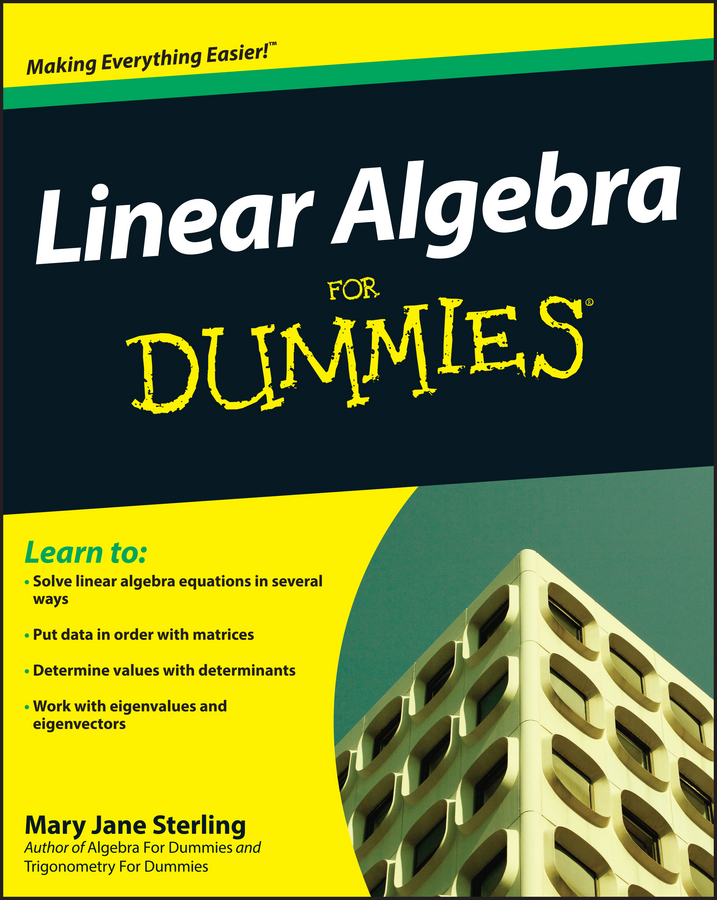##### Linear Algebra For DummiesThe factor theorem states that you can go back and forth between the roots of a polynomial and the factors of a polynomial. In other words, if you know one, you know the other. At times, your teacher or your textbook may ask you to factor a polynomial with a degree higher than two. If you can find its roots, you can find its factors.

In symbols, the factor theorem states that if xc is a factor of the polynomial f(x), then f(c) = 0. The variable c is a zero or a root or a solution — whatever you want to call it (the terms all mean the same thing).

Here’s an example. Say you have to look for the roots of the polynomial f(x) = 2x4 – 9x3 – 21x2 + 88x + 48. You find that they are x = –1/2, x = –3, and x = 4 (multiplicity two). How do you use those roots to find the factors of the polynomial?

The factor theorem states that if x = c is a root, (xc) is a factor. For example, look at the following roots:

• If x = –1/2, (x – (–1/2)) is your factor, which you write as (x + 1/2).

• If x = –3 is a root, (x – (–3)) is a factor, which you write as (x + 3).

• If x = 4 is a root, (x – 4) is a factor with multiplicity two.

You can now factor f(x) = 2x4 – 9x3 – 21x2 + 88x + 48 to get f(x) = 2(x + 1/2)(x + 3)(x – 4)2. Observe that 2 is a factor because 2 is the leading coefficient (the coefficient of the term with the highest exponent.)